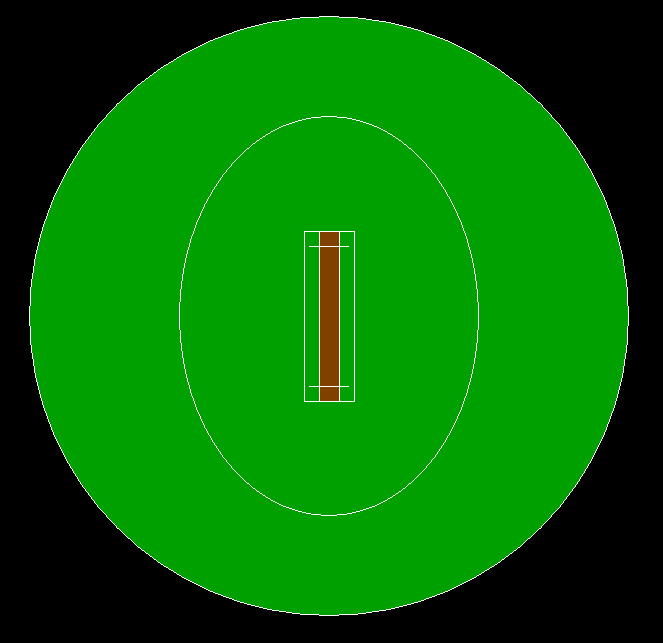# C program to draw a cricket ground using computer graphics

• Difficulty Level : Expert
• Last Updated : 04 May, 2021

In this article, we will discuss how to draw a 2D cricket ground is being designed using computer graphics.

Approach:

Below is the program for the above approach:

## C

 `// C program for the above approach`` ` `#include ``#include ``#include `` ` `// Driver Code``void` `main()``{``    ``int` `gd = DETECT, gm;`` ` `    ``// Initialize of gdriver with``    ``// DETECT macros``    ``initgraph(&gd, &gm, ``"C:\\turboc3\\bgi"``);`` ` `    ``// Ground Outline``    ``circle(700, 350, 300);`` ` `    ``// Coloring Green``    ``setfillstyle(SOLID_FILL, GREEN);``    ``floodfill(402, 350, 15);`` ` `    ``// 30 Yards Outline``    ``ellipse(700, 350, 0, 360, 150, 200);`` ` `    ``// Pitch Outer Line``    ``rectangle(675, 265, 725, 435);`` ` `    ``// Pitch Inner Line``    ``rectangle(690, 265, 710, 435);`` ` `    ``// Coloring Pitch Brown``    ``setfillstyle(SOLID_FILL, BROWN);``    ``floodfill(695, 300, 15);`` ` `    ``// Upper Stump Line``    ``rectangle(690, 265, 710, 280);``    ``line(680, 280, 720, 280);`` ` `    ``// Lower Stump Line``    ``rectangle(690, 435, 710, 420);``    ``line(680, 420, 720, 420);`` ` `    ``// Hold Screen For A While``    ``getch();`` ` `    ``// Close the initialized gdriver``    ``closegraph();``}`

Output:My Personal Notes arrow_drop_up